# Difference between revisions of "Tidal bore dynamics"

## Introduction

A tidal bore is a sudden elevation of the water surface that travels upstream an estuary with the incoming flood tide. This article describes the processes involved in this ultimate stage of tidal wave deformation and the modelling of these processes. For an introduction to the topic of tidal wave deformation the reader is referred to the article Tidal asymmetry and tidal basin morphodynamics.Figure 1. Left image : Partially breaking tidal bore on the Dordogne River (Courtesy of Antony “YEP” Colas). Right image : Undular tidal bore on the Garonne River (Bonneton et al. 2011 ).

Table 1. Estuaries and rivers with substantial tidal bores (bore height of half a meter up to several meters). The maximum tidal range is the maximum range recorded at a tide gauge situated close to the location in the estuary where the tidal range is highest.
Estuary/river name Maximum tidal range [m] Tide gauge location Country
Shannon River 5.6 Shannon Ireland
Humber,Trent 8.3 Hull UK
Great Ouse 7.4 King's Lynn
Dee 9.8 Flint
Mersey 10 Liverpool
Ribble 10.2 Lytham
Morecambe bay, River Kent 10.9 Morecambe
River Eden, Nith River 10.3 Silloth
Seine 8.5 Honfleur France
Canal de Carentan 7 Carentan
Baie du Mont Saint Michel – Sélune River 14 Granville
Garonne, Dordogne 6.3 Podensac
Pungue 7 Beira Mozambique
Qiantang 5 Ganpu China
Indus 4 Port Muhammad Bin Qasim Pakistan
Hooghly 5.7 Sagar Island India
Sittaung 6.3 Moulmein Myanmar
Batang Lupar River 5.6 Kuching Sarawak
Kampar River 5.3 Pulo Muda Sumatra
Hooghly 5.7 Sagar Island India
Fly , Bamu, Turamu , Mappi Rivers 4.2 estuary West Papua
Styx 6.4 Mackay, Queensland Australia
Daly River 7.9 estuary
Turnagain Arm, Knick Arm 7.9 Anchorage Alaska
Bay of Fundy, Petitcodiac and Salmon rivers 16 Truro Canada
Colorado River 7.7 San Filipe Mexico
Amazon, Araguira, Guama, Capim and Mearim Rivers 3.4 Macapa Brazil

## Occurrence of tidal bores

When a tidal wave propagates upstream into an estuary, its shape is progressively distorted. In many estuaries the high-water (HW) tidal wave crest propagates faster up-estuary than the low-water (LW) tidal wave trough. HW propagates considerably faster than LW if the mean channel depth $D_0$ is not much greater than the spring tidal range $2a$ (same order of magnitude or a few times larger) and if the intertidal area is not much greater than the tidal channel surface area. The tidal rise period is shortened and the tidal wave becomes steeper during propagation. If the tidal wave can propagate sufficiently far upstream the river without strong damping, the tidal wave front may become so steep that a water level jump – a so-called tidal bore - can form at the beginning of the rising tide.

Table 1 presents estuaries and tidal rivers in which significant tidal bores have been observed (a more complete overview can be found in Bartsch-Winkler and Lynch (1988)  and a recently updated catalog by Colas (2017)). It appears that tidal bores generally occur for large tidal ranges ($Tr_0$) at the estuary mouth. However, such a simple criterion is not sufficient to classify estuaries in terms of tidal bore occurrence. For instance, Furgerot (2014)  showed that in the Sée/Mont Saint Michel estuary, $Tr_0$ must be larger than 10 m for tidal bore formation in the Sée River and, on the other hand, Bonneton et al. (2015) observed tidal bores in the Gironde/Garonne estuary even for $Tr_0$ smaller than 2 m.

The estuarine shape (depth and width profiles) plays an important role in tidal bore formation. This is illustrated by the observation that regulation works (dredging, reclamation, weirs) during the past century have weakened or even completely suppressed tidal bores in many rivers, for example in the rivers Seine, Charente, Colorado and Petitcodiac. We will discuss in the following general conditions for bore formation in terms of estuary geometry and flow conditions. g

Illustrations of tidal bores are shown in Fig. 1.

## Tidal bore formation in funnel-shaped estuaries

The analysis of nonlinear tidal wave transformation in estuaries, in terms of tidal forcing at the estuary mouth and large-scale geometrical properties of the channel, has received considerable attention (see Tidal asymmetry and tidal basin morphodynamics). By contrast, the extreme nonlinear tidal-wave case where tidal bores form is much less studied.

The most intense tidal bores occur in long and shallow tide-dominated funnel-shaped estuaries (e.g. Qiantang, Severn, Kampar, Gironde/Garonne/Dordogne, …) . The formation of these tidal bores is governed by the progressive distortion of the tidal wave as it propagates up the estuary. If the ebb-flood asymmetry (duration of falling tide longer than rising tide and larger flood than ebb currents) is strong enough, a tidal bore can form in the upper estuary. This is illustrated by the tidal asymmetry in the Garonne at Bordeaux (Fig. 2) and the upstream tidal bore at Podensac (Fig.3).

This extreme nonlinear deformation of a tidal wave occurs in a limited number of estuaries. To determine the conditions favourable to tidal bore occurrence, a scaling analysis can be carried out for a schematic channel geometry. A usual schematization is based on the approximations :

• Uniform channel depth $D$,
• Exponential convergence of the width $B(x)$.

The convergence length $L_b$, defined as $L_b=B(x)/dB/dx$, is assumed independent of the along-channel coordinate $x$. The forcing tidal wave at the estuary mouth can be characterized by its tidal frequency $\omega=2 \pi / T$ and its amplitude $A_0=Tr_0/2$, where $Tr_0$ is the estuary mouth tidal range. Another important parameter which influences tidal propagation in an estuary is the dimensionless mean friction coefficient $c_D$. The tidal wave dynamics is then controlled, if we neglect fresh water discharge effects, by three dimensionless parameters:

• the nonlinearity parameter, $\epsilon=A_0/D$,
• the friction parameter, $\phi=c_D \sqrt{gD}/(\omega D)$,
• the convergence ratio, $\delta=\sqrt{gD}/(\omega L_b)$.Figure 2. Tidal wave distortion and bore formation observed in the Gironde-Garonne estuary (Bonneton et al. 2015) at spring tide. Left panel: Tide gauge locations on the estuary. Right top panel: Time series of tide elevation at 4 different locations, from the estuary mouth (Le Verdon) to the upper estuary (Podensac); Right bottom panel: Tidal bore illustrations at Podensac field site, located 126 km upstream the river mouth.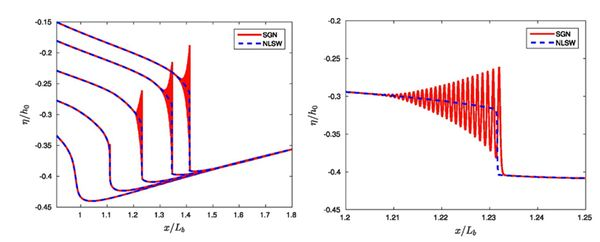Figure 3. Tidal wave distortion and bore formation in a convergent estuary. Numerical simulations by Filippini et al. 2019 . Left panel: Rising tide elevation at different increasing times. Right panel: Zoom of left panel.

The formation of tidal bores is mainly governed by the progressive distortion of the tidal wave as it propagates up the estuary. This extreme nonlinear deformation of the tidal wave occurs under special conditions, in particular :

• a large tidal amplitude $a$,
• a long, shallow and convergent channel.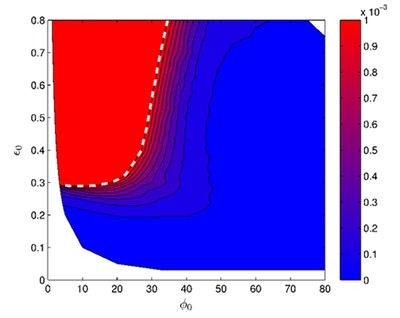Figure 4. Estuarine classification in parameter space ($\epsilon, \phi$). Maximum surface elevation slope $A_{max}$, the white dashed line represents the $\epsilon_c$ curve, namely the limit for tidal bore appearance following the criterion $A_{max} \ge 10^{-3}$. Each point in the plane ($\epsilon, \phi$) represents a numerical tidal wave solution for an idealized convergent estuary; this figure relies on 225 numerical simulations (Filippini et al., 2019).

Available field data suggest that tidal bores form in convergent estuaries characterized by large values of the convergence ratio $\delta$. Bonneton et al. (2016) and Filippini et al. (2018) have analysed tidal bore occurrence as a function of the dimensionless parameters $\epsilon$ and $\phi$ for a fixed value of the convergence ratio $\delta=2.4$. Fig. 4 presents the position of convergent estuaries in the parameter space ($\epsilon, \phi$). We can observe a clear separation between tidal-bore estuaries and non tidal-bore estuaries. Tidal bores occur when the nonlinearity parameter $\epsilon$ is greater than a critical value, $\epsilon_c$, which is an increasing function of the friction parameter $\phi$. For small $\phi$-values (friction parameter $\phi \approx 15$), tidal bores can form for $\epsilon$ greater than 0.2. By contrast, for large $\phi$-values the tidal bore formation requires much larger nonlinearity parameters. These results show that bore formation is mainly controlled by the competition between two physical processes: (a) the nonlinear distortion of the tidal wave, which is favourable to bore inception; (b) the friction dissipation of the tidal wave, which is unfavourable to bore formation.

## Conditions for tidal bore formation

More generally, the analysis of tidal bores observed in natural estuaries suggests the following conditions for tidal bore formation.

The first requirement for tidal bore formation is sufficient length of the estuarine-tidal river system, i.e. the tide can penetrate far enough into the system. This depends primarily on the average longitudinal slope of the estuary-tidal river system, with a secondary role for the convergence length $L_b$. A steep slope limits the penetration of the tide. A small channel slope is generally indicative of low-to-moderate current velocities and friction (steep slopes are generally related to strong friction generated by high current velocities and coarse bed sediments). If the longitudinal channel slope is small, the tidal bore can travel over a long distance without much dissipation.

The tidal bore develops in a section of the estuary-tidal river system where the nonlinearity parameter $\epsilon$ is large (shallow depth, large tidal range) and where the tidal wave is flood-dominant. Sometimes a tidal bore already forms close to the mouth of the estuary. This is the case with estuaries that have a large mouth bar, such as the Qiangtang estuary and the Charente estuary (the latter before large-scale interventions took place). But this is rather the exception. Most often the tidal bore is formed in a section of the estuary-tidal river system further upstream, in the tidal river or on the tidal flats at the head of the estuary (as for the Garonne, Seine, Sée, etc.).

The condition of a large tidal range and a strong tidal asymmetry depends on friction; one should therefore distinguish between muddy and sandy/gravelly estuaries. If the solid fluvial flow is important with a large fraction of cohesive mud (mainly estuaries in the (sub) tropic regions, but also the Garonne) the estuarine channel bed is smooth so that the friction is weak (small $\phi$); the tidal wave is not strongly damped. In this case a small relative channel depth (large $\epsilon$) is the main condition to obtain a strong asymmetry of the tidal wave. Rivers with low solid discharge or supplying sandy/coarse sediments have a sandy or gravelly channel bed in the estuarine zone that produces strong friction (large $\phi$) and strong tidal energy dissipation. In this case, strong convergence of the width of the estuary (large $\delta$) is required to generate a large, highly asymmetric tidal amplitude (large $\epsilon$).

River flow also influences tidal bore formation. River flow contributes to tidal wave deformation by enhancing the longitudinal velocity gradient. However, river flow also contributes to tidal wave damping and thus opposes tidal bore formation . The latter effect usually dominates. Observations show that the tidal bore in the Garonne and Dordogne (France) is suppressed at high river runoff. For large discharge (order of 1000 m3/s), tidal bores do not form. By contrast, for low discharge (order of 150 m3/s) a tidal bore almost always occurs in the Garonne River, even at neap tide. These findings were confirmed by Filippini et al. (2019) from numerical simulations. A similar effect is observed in the Daly estuary by Wolanski et al. (2004), who relate the occurrence of the tidal bore at low river discharge to the small water depth during such conditions. However, the opposite effect is reported for the Guamá-Capim river system near the mouth of the Amazon River, where tidal bores are observed only at high river discharges, in conjunction with high equinoctial tides.

Variations in local river geometry and water depth can also significantly affect tidal bore dynamics (Bonneton et al. (2011) and Keevil et al. (2015)). Observations show that tidal bores often develop in shallow rivers that discharge from the higher upstream zone into a broad estuary. If the tidal wave has already acquired sufficient asymmetry when travelling through the estuary, a tidal bore develops when the tidal wave surges into the shallow river. The presence of a mouth bar (large $\epsilon$) is favourable to the development of strong asymmetry of the tidal wave and tidal bore formation, see Fig. 5. On the contrary, the presence of local channel width constrictions (large $\phi$) is unfavourable to tidal bore formation, as illustrated by the absence of a tidal bore in the Conway estuary in spite of a high non-linearity parameter.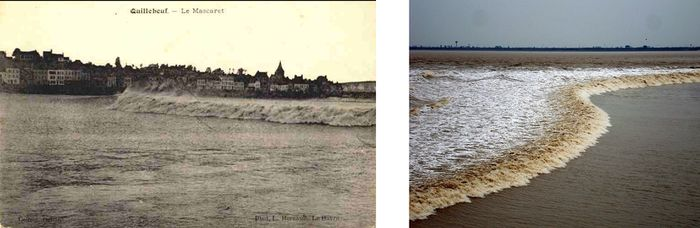Figure 5. Many natural estuaries have large shoals in the mouth zone. These so-called mouth bars strongly enhance distortion of the tidal wave entering the estuary. Left image: Tidal bore in the Seine River around 1960, before the mouth bar was dredged. Right image: Tidal bore in the Qiangtang estuary, where a large mouth bar is still present (https://en.wikipedia.org/wiki/Qiantang_River ).

## Tidal bore characteristics

A tidal bore can be schematically represented by a propagating transition between two streams of water depth $D_1$ and $D_2$, with $D_1 \lt D_2$ (see Fig. 6). Once such a hydraulic jump has developed, its flow velocities ahead and behind the jump can be derived from the mass and momentum balance equations (Fig. 6):Figure 6. Schematic representation of a hydraulic jump propagating with velocity $c$. In the frame moving with velocity $c$ the hydraulic jump appears stationary; time derivatives are zero. The corresponding mass and momentum balance equations from which the propagation characteristics can be derived are indicated in the figure. The symbols used stand for: $u$= flow velocity in a fixed frame, $v$= flow velocity in the moving frame, $D$= water depth, $g$=gravitational acceleration; subscripts 1 and 2 indicate upstream and downstream conditions, respectively.

$v_1=u_1 – c = - \Large \sqrt{ \frac{g D_2 (D_2 + D_1)}{2 D_1}} \normalsize , \quad \quad (1)$

$v_2=u_2 – c = - \Large \sqrt{ \frac{g D_1 (D_2 + D_1)}{2 D_2}} \normalsize , \quad \quad (2)$

where $c$ is the bore celerity, $v$ the velocity in the moving frame and $u$ in the fixed frame. These equations show that the problem is entirely determined by the depth ratio $D_2 / D_1$, or equivalently by $\Delta D / D_1$, where $\Delta D = D_2 – D_1$ is the bore height. The Froude numbers around the jump, in the moving frame can be obtained from equations (1) and (2):

$F_1^2 = \Large \frac{v_1^2}{g D_1}\normalsize = \large \frac{D_2 (D_1+D_2)}{2 D_1^2} \normalsize , \quad \quad (3)$

$F_2^2 = \Large \frac{v_2^2}{g D_2}\normalsize = \large \frac{D_1 (D_2+D_1)}{2 D_2^2} \normalsize . \quad \quad (4)$

Knowing that $D_2 \gt D_1$, it is straightforward to see that $F_1 \gt 1$ and $F_2 \lt 1$. The jump correspond to the passage from a supercritical flow ($F_1 \gt 1$) to a subcritical flow ($F_2 \lt 1$). The bore intensity is characterized equally by $\Delta D / D_1$ or by the Froude number $F_1$.

If $F_2 = 1$ (the bore height $\Delta D$ is zero) the bore velocity $c$ is equal to

$c_2 = u_2 + \sqrt{g D_2}$.

The inequality $F_2 \lt 1$ therefore implies $c \lt c_2$. Because $c_2$ is the propagation speed of long-wave disturbances upstream of the bore, these disturbances (undulations) will catch up to the bore. A slowly propagating bore ($F_2 \lt \lt 1$) will thus grow faster and become higher than a fast propagating bore ($F_1, F_2$ close to 1) .

It can be shown that conservation of mass and momentum at the transition does not imply conservation of energy. From the mass and momentum equations the following expression for the energy dissipation is obtained  :

$Q \Delta E = D_1 V_1 [ \rho g \Delta D + 0.5 \rho (v_2^2 -v_1^2) ] = \Large \frac{\rho g}{4} \sqrt{\frac{g (D_1+D_2)}{2 D_1 D_2}}\normalsize (D_2 – D_1)^3 , \quad \quad (5)$

Two forms of energy dissipation can occur at the transition, leading to two different types of bores: undular and turbulent bores.

### Undular bores

For $F_1$ smaller than approximately 1.3, the bore transition is smooth and followed by a wave train (Fig. 1 right). The bore then consists of a mean jump between two water depths (see Fig. 6) on which secondary waves are superimposed. This type of bore is usually called an undular bore and Favre (1935)  was the first to describe this phenomenon from laboratory experiments. That is why undular bores are sometimes referred to as Favre waves. This phenomenon is a dispersive wave process (http://www.coastalwiki.org/wiki/Dispersion_(waves)), which is also named “dispersive shock” in the mathematical community.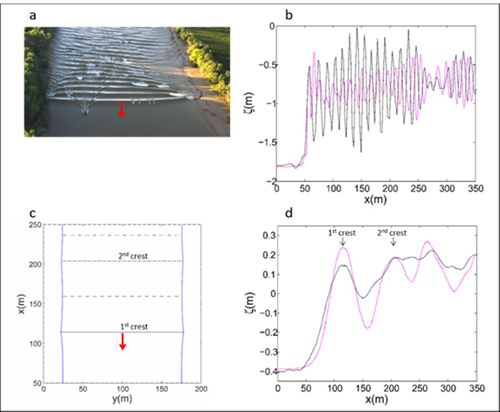Figure 7. Illustration of the two undular tidal bore regimes (Garonne River, Bonneton et al. 2015). Panels a, b: Dispersive regime (“mascaret” in French), F1=1.27. Panels c, d: Dispersive-like regime, Fr=1.08. Black line: elevation in the mid-channel; magenta line: elevation close to the river bank. Panel a:Two-dimensional (2-D) phase structure. Panel c: Quasi-1D phase structure.

A simple model describing the secondary waves attached to the bore was proposed by Lemoine (1948) . He considered that secondary waves can be approximately described by linear theory. The propagation speed in the fixed frame is, according to linear shallow-water wave theory,

$c_w = u_2 + \sqrt{gD_2 (1- \frac{k^2 D_2^2}{3})} , \quad \quad (6)$

where $k=2 \pi / \lambda$ is the wave number and $\lambda$ the wavelength. The secondary waves are stationary in a frame moving with the bore front, i.e. $c_w = c$. This equality provides an estimate of the wavelength:

$\lambda=\sqrt{2/3} \pi D_2 (F_1 – 1)^{-1/2} . \quad \quad (7)$

The secondary waves are stationary in the frame moving with the bore front, but in this frame wave energy is radiated behind the front. Equating the energy flux with the mean bore dissipation (Eq. 5) gives the secondary wave amplitude as a function of $F_1$:

$a_w=\frac{4}{3\sqrt{3}} D_2 (F_1 – 1) . \quad \quad (8)$

As this approach ignores wave-induced mass transport it is valid only for very small bores ($F_1$ close to 1). Nevertheless, equations (7) and (8) appear to give a correct estimate of the observed wavelength and amplitude of undular bore propagating in rectangular channels .Figure 8. Numerical simulations of the two undular tidal bore regimes. Panel a: Dispersive regime, F1=1.20. Panel b: Dispersive-like regime, F1=1.05. From Chassagne et al. 2019.

In reality, natural estuary and river channels are non-rectangular and present most of the time a variable cross-section with an approximately trapezoidal shape and gently sloping banks. The propagation of undular bores over channels with variable cross-sections was studied by Treske (1994) in the laboratory and by Bonneton et al. (2015) in the field. Both studies identified a transition around $F_t=1.15$. For $F_1 \gt F_t$ the secondary wave field in the mid channel is very similar to the dispersive waves (Favre waves) described above, and follow the relations (7) and (8). For $F_1 \lt F_t$, the secondary wave wavelength in the whole channel is at least two to three times larger than in a rectangular channel for the same Froude numbers. It was shown that this new undular bore regime (Figs. 7 c,d and 8 b) differs significantly from classical dispersive undular bores in rectangular channels (i.e. Favre waves). Chassagne et al. (2019)  recently showed that this undular bore regime (named dispersive-like bore) is controlled by hydrostatic non-dispersive wave properties, with a dynamics similar to edge-waves in the near-shore. The transition between dispersive and dispersive-like bores is illustrated on Fig. 7 and 8. The dispersive-like bores are characterized by low wave steepness, which make them difficult to visually observe in the field. This is why such tidal bores are generally ignored and why tidal bore occurrence in the field is generally underestimated  .

### Breaking bores

For large Froude numbers, bores correspond to turbulent breaking fronts (Fig. 5), where the energy is dissipated by turbulent eddies. The transition between undular and turbulent bores is shown in Fig. 11. Before arrival of the tidal bore the current velocity is generally low and in the ebb direction. At the arrival of the bore front the current velocity is almost instantaneously reversed into the flood direction and reaches a high value within minutes. Fig. 9 shows the sharp increase in the current velocity and suspended sediment concentration recorded in the megatidal Baie du Mont Saint Michel at spring tide when the tidal flood wave enters the tidal flat area and the small rivers at the head of the Baie . The sudden strong change of the current velocity has an important stirring effect on the bed sediments, causing a sharp increase of the suspended sediment concentration. Turbulent bores contribute significantly to upstream sediment transport.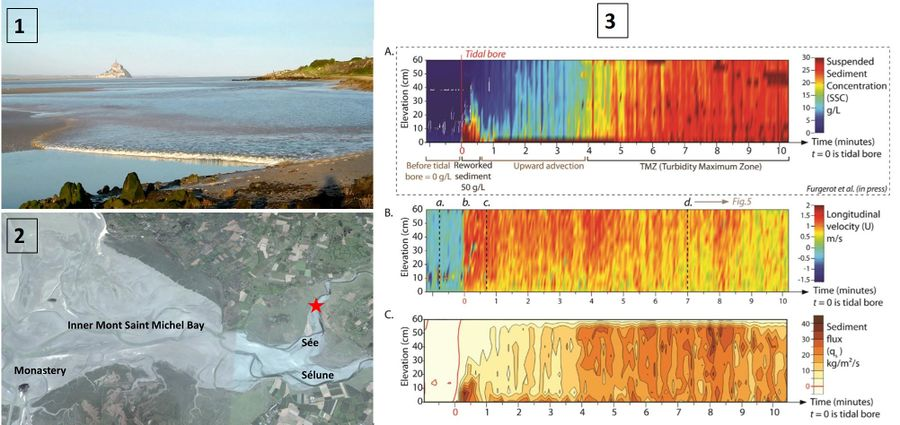Figure 9. Measurement of sediment transport by a tidal bore in the Sée River on 8 May 2012 (Furgerot et al, 2016 ). Panel 1: Tidal bore entering the Sée channel at the head of the Baie du Mont Saint Michel. The monastery is visible at the background. Panel 2: Map of the inner part of the Baie du Mont Saint Michel. The measuring location in the Sée River is indicated by the red star. Panel 3: Temporal variation of the vertical distribution of suspended sediment concentration (top), current velocity (middle) and sediment flux just (bottom) before and after arrival of the tidal bore. The current is ebb-directed with very low velocity just before arrival of the tidal bore and reverses to a flood-directed velocity simultaneously with the arrival of the tidal bore (middle panel). A very high short peak in the suspended sediment concentration near the bed occurs at the passage of the tidal bore front. High suspended sediment concentrations over the entire vertical (mainly fine silty sand) are recorded about 5 minutes later representing the sediment advected by the tidal bore (top panel). After a very high peak at the passage of the bore front the sediment flux is again high and in up-river direction some minutes later. The sediment flux is much lower during the remaining flood period (bottom panel) and similar to the up-river sediment flux when a tidal bore is absent. A video of the arrival of the tidal bore can be viewed by clicking here. The photo of panel 1 is a still from the video.

In many tidal rivers the bore propagation near the shallow channel banks differs from the bore propagation in the deeper middle part of the channel. The local Froude number is relatively lower at the deeper parts of the channel, where the bore has often an undulating character, while a higher breaking bore occurs in the shallower parts. This phenomenon is illustrated in Fig. 10 for the tidal bore in the Petitcodiac and Kampar rivers.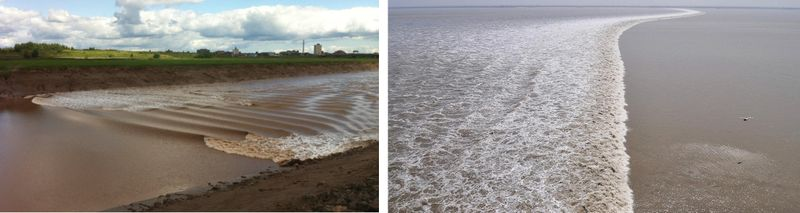Figure 10. Left image: Tidal bore in the Petitcodiac River, which is undular at the middle of the channel and breaking near the channel banks. Right image: Breaking tidal bore in the Kampar River, Sumatra.

## Modelling

Tidal bore formation involves a large range of temporal and spatial scales, from the scale of the estuary to the turbulence scale. For this reason, it is difficult to integrate all these processes into a comprehensive model, either a physical model (laboratory experiments) or a numerical model.

### Physical models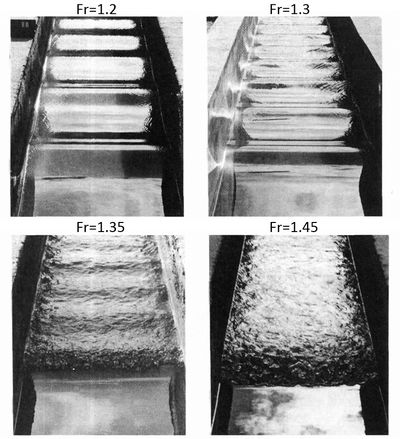Figure 11. Experimental study of undular bores (Favre-waves) in open rectangular channels (Treske 1994<name=Tr></ref>). Undular bores, Fr=1.2 and 1.3; transition to a turbulent bore, Fr=1.35; turbulent bore, Fr=1.45.

Due to the large range of scales, it is impossible to design a laboratory experiment in close similitude with natural tidal bores. However, leaving aside the tidal wave transformation and bore formation, the bore in itself (i.e. hydraulic jump in translation) can be studied in detail from flume experiments. The bore is commonly generated in a rectangular flume by using a fast-closing gate at the upstream end of the flume. This method allows the study of the different bore regimes (see Fig. 11) and provides valuable insights in secondary wave structure and vortical motions.

To avoid the abrupt bore generation of the above method, Rousseaux et al. (2016) proposed a novel approach. This method mimics the tidal asymmetry met in nature between the ebb and the flood. Fig. 12 shows an example of a tidal-like bore generated with this method.Figure 12. Experimental study of tidal-like bore using a laser sheet and fluorescent dye (Rousseaux et al. 2016).

### Numerical modelsFigure 13. Large Eddy Simulation of turbulence generated by a weak breaking bore. Streamlines indicate recirculation structures under the bore (Lubin et al. 2010).

Recent approaches, based on the resolution of the general Navier Stokes equations in their multiphase form (water phase and air phase), allow a detailed description of bore structure, turbulence and air entrainment in a roller at the bore front. Figure 13 presents the simulation of recirculating structures under a breaking bore. Navier-Stokes approaches are dedicated to small scale bore processes and cannot be applied to tidal bores at the estuarine scales because of limited computation power. Such applications would require long-wave models, where small scale vorticity motions are parametrized and not directly resolved.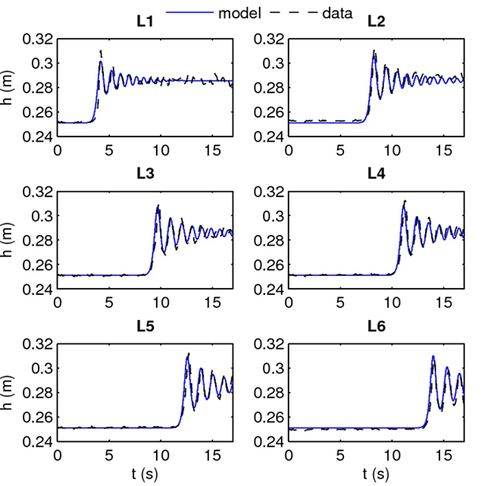Figure 14. Serre-Green Naghdi simulation of an undular bore. Comparisons between experimental data (at 6 gauges, Fr=1.104) from Soares-Frazao and Zech (2002)  and model prediction (Tissier et al. 2011).

The most common long-wave model is the depth-averaged Saint Venant or Non Linear Shallow Water (NSW) model, which assumes hydrostatic pressure. This model gives a good description of the large-scale tidal wave transformation. The properties of breaking turbulent bores, are also quite well described by the non-dispersive NSW equations with jump conditions . However, the onset of a tidal bore and its evolution upstream is controlled by non-hydrostatic dispersive mechanisms. Although the onset of the tidal bore can be well described by classical weakly dispersive weakly nonlinear Boussinesq-type equations (, see Boussinesq equations), the subsequent nonlinear evolution, for high-intensity tidal bores, requires the use of the basic fully nonlinear Boussinesq equations, named Serre-Green Naghdi (SGN) equations. This modelling approach allows an accurate description of both the tidal bore formation on the estuarine scale (Fig. 4) and the bore structure on the local scale (Fig. 14).